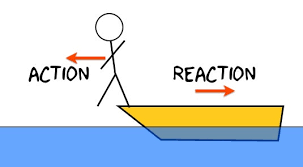top of page
Search

# Newton's Laws of Motion: Relationship between force and object

Imagine a world with practically no development, where items stay fixed and undisturbed. This vision of tranquility stands out forcefully from the powerful reality we possess. From the effortless trip of birds to the taking off send off of rockets, the laws of movement oversee each part of our actual presence. What's more, at the core of this understanding reveals Sir Isaac Newton's notable laws of movement. In this blog, we will jump into these essential rules that have enlightened the mechanics of our universe.

First Law of Motion:

Newton's most memorable law of movement, called the law of Inertia, makes way for grasping the way of behaving of articles very still and moving. It expresses that an article will stay very still or keep moving in an orderly fashion at a consistent speed except if followed up on by an outer force. Generally, this law highlights the idea that objects normally oppose changes in their condition and direction of movement.

Newton's 1st Law states that:

An object at rest stays at rest and an object in motion stays in motion at a constant speed and direction unless acted upon by an unbalanced force.

Motion occurs when equilibrium is disturbed by the interference of additional force, hence net force is zero and causes the object to perform motion.First Law of Motion

In the above figure, ball is in the ground with net force equals to zero. Gravity has cancelled the ground's up thrust force but force from left hand side might be the kick from the footballer will make the ball move in the right direction so until an unless external force applies on the object, object will stay at its position like book lying on the table unless any force make it fall from the table , frame hanging on the wall unless wind(force) tilts it.

Newton's second law of motion:

This law states:

The acceleration of the body is directly proportional to the net force acting on the body and inversely proportional to the mass of the body.

F is directly proportional to acceleration

Acceleration a body attains is inversely proportional to the mass of it's body

Therefore, F=ma, where F and a are vectors.

This law interpret that as you are pushed with greater force, you'll move faster and bigger the mass of the body, slowly it will move.Second Law of Motion

Above figure summarizes the second law and says that to move/accelerate it faster, higher force is required and if the mass is higher, more force is required to accelerate it.

Newton's Third Law of Motion:

This law states that "For every action there is an equal and opposite reaction" OR

"Forces always occur in pairs. The two forces are of equal strength, but in opposite directions."

If object A is exerting force on object B then in response, object B will also exert force on object A. These forces are considered to cancel each other but they do not. Forces act on two different bodies or systems that's why they don't cancel each other. This law is also called Action-reaction. Reaction is made when action is done.

Newton's 3rd Law exhibits many examples.Third Law of Motion

In this figure, a boy is acting force on boat in order to move forward and jump whereas boat exert direction on the boy in forward direction to help him jump. He applied force on the boat in the opposite direction of the direction in which he has to jump. These two forces were acted on two different bodies that's why they don't cancel each other.

Conclusion: Newton's laws of motion are those three basic laws that describe relationship between motion of an object and forces acting on it. They can be interpreted as follows:

A body will remain at rest unless an external force acts upon it. A body's acceleration is directly proportional to the force acting upon it and inversely proportional to the mass of the body. For every action, there is an equal and opposite reaction.